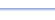Program: LINES

LINES  (free version available here)

• Finds the equation of a line, given any of the following pieces of information:  slope and y-intercept, slope and point, 2 points, x-intercept and y-intercept, point and slope of parallel line, point and slope of perpendicular line.
• Changes the equation of a line from slope-intercept form to standard form or the reverse.

Useful for:

• SAT
• ACT
• Algebra 1
• Algebra 2
• Geometry
• Calculus

See full list of programs

Try these problems using LINES:
on MathPro Q&A Forum(Free version has options 1-3 only.)
Example 1

Q: Find the equation of the line that passes through the points (6,-3) and (2,1).

Solution:

Run LINES.  How?

Select choice 3.

Type each number
as follows, pressing
ENTER after each one:

The program reports:

Interpretation:   y = -x + 3  or  x + y = 3

See Example 2